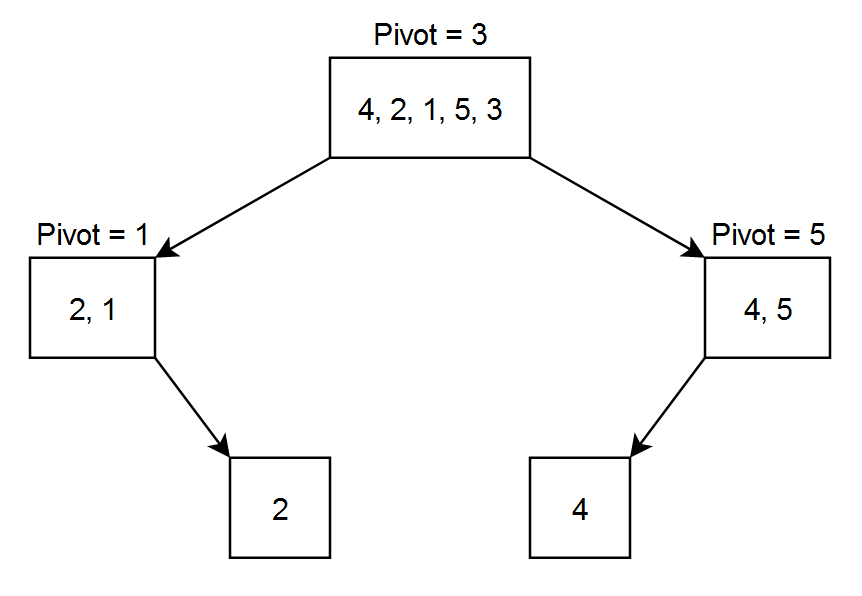3

# Quick Sort

Difficulty: MEDIUMContributed By
Anup Kumar Singh
Avg. time to solve
10 min
Success Rate
90%

Problem Statement

#### Example:

``````Let the array = [ 4, 2, 1, 5, 3 ]
Let pivot to be the rightmost number.
``````#### After the 1st level partitioning the array will be { 2, 1, 3, 4, 5 } as 3 was the pivot. After 2nd level partitioning the array will be { 1, 2, 3, 4, 5 } as 1 was the pivot for the left part and 5 was the pivot for the right part. Now our array is sorted and there is no need to divide it again.

##### Input format:
``````The first line of input contains an integer 'T' denoting the number of queries or test cases.

The first line of each input consists of an integer 'N' denoting the size of the array.

The second line of each input consists of 'N' space-separated integers denoting the elements of the array.
``````
##### Output format:
``````For each test case, print a single line containing space-separated integers denoting the elements of the array after sorting.

The output of each test case will be printed in a separate line.
``````
##### Note:
``````You do not need to print anything, it has already been taken care of. Just implement the given function.
``````
``````Can you solve this in the worst case NlogN complexity?
``````
##### Constraints:
``````1 <= T <= 10
1 <= N <= 10 ^ 3
-10 ^ 9 <= ARR[i] <= 10 ^ 9

Where 'T' is the number of test cases, 'N' is the length of the array 'ARR', and 'ARR[i]' is the array element at index i.

Time limit: 1 sec.
``````
##### Sample Input 1:
``````2
5
4 2 5 1 3
4
6 2 4 1
``````
##### Sample Output 1:
``````1 2 3 4 5
1 2 4 6
``````
##### Explanation of Input 1:
``````Test case 1:
Given array =  [4, 2, 5, 1, 3]
Sorted array = [1, 2, 3, 4, 5]

Test case 2:
Given array =  [6, 2, 4, 1]
Sorted array = [1, 2, 4, 6]
``````
##### Sample Input 2:
``````2
5
5 3 2 6 4
4
1 2 3 4
``````
##### Sample Output 2:
``````2 3 4 5 6
1 2 3 4
``````Console